# MP Board Class 8th Maths Solutions Chapter 13 Direct and Inverse Proportion Ex 13.2

## MP Board Class 8th Maths Solutions Chapter 13 Direct and Inverse Proportion Ex 13.2

Question 1.
Which of the following are in inverse proportion?
(i) The number of workers on a job and the time to complete the job.
(ii) The time taken for a journey and the distance travelled in a uniform speed.
(iii) Area of cultivated land and the crop harvested.
(iv) The time taken for a fixed journey and the speed of the vehicle.
(v) The population of a country and the area of land per person.
Solution:
(i) If the number of workers on a job increases (decreases), then the time to complete the job will decrease (increase).
∴ The given statement is in inverse proportion.
(ii) If the time taken for a journey increases (decreases), then the distance travelled in uniform speed will also increase (decrease).
∴The given statement is in direct proportion.
(iii) If the area of cultivated land increases (decreases), then the crop harvested will also increase (decrease).
∴ The given statement is in direct proportion.
(iv) For a fixed journey, if the speed of the vehicle increases (decreases), then the time taken for journey will decrease (increase).
∴ The given statement is in inverse proportion.
(v) If the population of a country increases, then the area of land per person will decrease. But if the population of a country decreases, then the area of land per person will increase.
∴ The given statement is in inverse proportion.Question 2.
In a Television game show, the prize money of ₹ 1,00,000 is to be divided equally amongst the winners. Complete the following table and find whether the prize money given to an individual winner is directly or inversely proportional to the number of winners?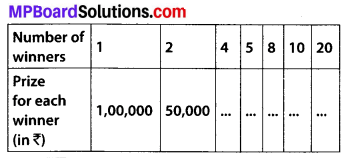Solution:
Since, we know that if the money is to be distributed in more and more people, then the amount of money given to an individual will decrease i.e., the given problem is in inverse proportion.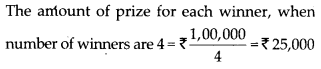The amount of prize for each winner, when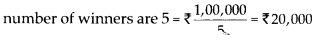The amount of prize for each winner, when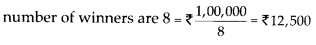The amount of prize for each winner, whenThe amount of prize for each winner, whenQuestion 3.
Rehman is making a wheel using spokes. He wants to fix equal spokes in such a way that the angles between any pair of consecutive spokes are equal. Help him by completing the following table.(i) Are the number of spokes and the angles formed between the pairs of consecutive spokes in inverse proportion?
(ii) Calculate the angle between a pair of consecutive spokes on a wheel with 15 spokes.
(iii) How many spokes would be needed, if the angle between a pair of consecutive spokes is 40°?
Solution:
If the number of spokes increases, then the angle between them will decrease.
∴ The given problem is in inverse proportion. Then angle between a pair of consecutive spokes,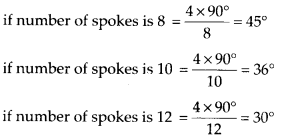(i) Yes, they are in inverse proportion.
(ii) The angle between a pair of consecutive spokes on a wheel with 15 spokes $$=\frac{4 \times 90^{\circ}}{15}=24^{\circ}$$
(iii) Number of spokes, if the angle between a pair of consecutive spokes is 40°
$$=\frac{4 \times 90^{\circ}}{40^{\circ}}=9$$Question 4.
If a box of sweets is divided among 24 children, they will get 5 sweets each. How many would each get, if the number of the children is reduced by 4?
Solution:
Let the number of sweets, each child would get be x.
According to question,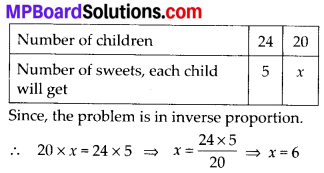Therefore, each child would get 6 sweets.Question 5.
A farmer has enough food to feed 20 animals in his cattle for 6 days. How long would the food last if there were 10 more animals in his cattle?
Solution:
Let the food would last for x days.
According to question,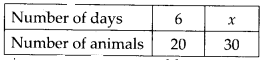Since, the given problem is in inverse proportion.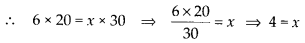Therefore, the required number of days is 4.Question 6.
A contractor estimates that 3 persons could rewire Jasminder’s house in 4 days. If he uses 4 persons instead of three, how long should they take to complete the job?
Solution:
Let the number of days = x
According to question,Therefore, 4 persons will take 3 days to complete the job.

Question 7.
A batch of bottles were packed in 25 boxes with 12 bottles in each box. If the same batch is packed using 20 bottles in each box, how many boxes would be filled?
Solution:
Let the number of boxes to be filled = x
According to question,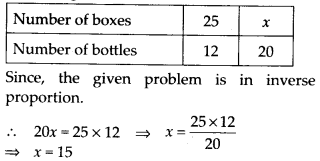Therefore, the required number of boxes to be filled would be 15.Question 8.
A factory requires 42 machines to produce a given number of articles in 63 days. How many machines would be required to produce the same number of articles in 54 days?
Solution:
Let the number of machines required to produce the articles in 54 days = x
According to question,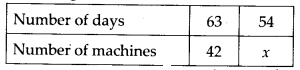If number of days are decreasing, number of machines must be increasing.
∴ The given problem is in inverse proportion.Therefore, the number of machines required to produce the same number of articles in 54 days = 49

Question 9.
A car takes 2 hours to reach a destination by travelling at the speed of 60 km/h. How long will it take when the car travels at the speed of 80 km/h?
Solution:
Let the time taken by car at the speed of 80 km/h = x hours
According to question,If the speed of a car increases, then the time taken by the car will decrease.
∴ The given problem is in inverse proportion.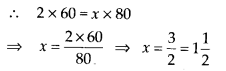Therefore, time taken by car is 1$$\frac{1}{2}$$ hours.Question 10.
Two persons could fit new windows in a house in 3 days.
(i) One of the persons fell ill before the work started. How long would the job take now?
(ii) How many persons would be needed to fit the window in one day?
Solution:
(i) Suppose 1 person finishes the work in x days.
According to question,If the number of workers/persons will decrease, then present workers/persons will take more time to finish the job.
∴ The given problem is in inverse proportion.
∴ 2 × 3 = 1 × x ⇒ x = 6
Therefore, one person will take 6 days to finish the job.

(ii) Let the number of persons required to fit the window in one day = x
According to question,Therefore, 6 persons are needed to finish the work in one day.Question 11.
A school has 8 periods a day each of 45 minutes duration. How long would each period be, if the school has 9 periods a day, assuming the number of school hours to be the same?
Solution:
Let the duration of each period be x minutes.
According to question,If the number of periods increases, then the time duration of each period will decrease.
∴ The problem is in inverse proportion.Therefore, each period would be 40 minutes long.